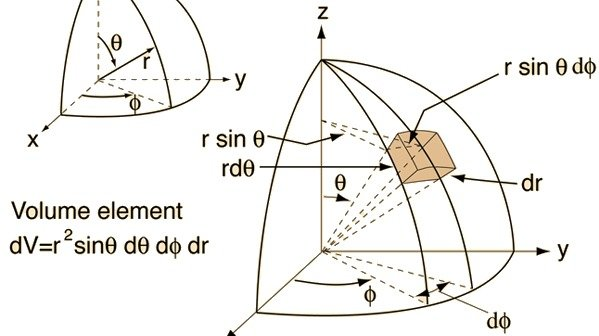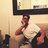Petition Closed# Create a unified notation for the spherical coordinates in space.Say you are confused about spherical coordinates, and you Google it to get a grasp of how it works. Your lecturer specified θ to be the "angle measured from the z-axis to the r-vector" and φ to be the "azimuthal angle, or angle measured from the x axis to the projection of the r-vector onto the xy-plane".

However, these two angles are often swapped online, as these images show:

http://mathworld.wolfram.com/images/eps-gif/SphericalCoordinates_1201.gif

http://hyperphysics.phy-astr.gsu.edu/hbase/Math/immath/sphcoordel.gif

https://qph.ec.quoracdn.net/main-qimg-9d30b330dc430ca6c396acc0953080cf

This yields further confusion when discussing the relationship between Cartesian coordinates and spherical coordinates, as well as when defining the nabla operator in spherical coordinates.

"Unfortunately, the convention in which the symbols θ and φ are reversed (both in meaning and in order listed) is also frequently used, especially in physics. This is especially confusing since the identical notation (r,θ,φ) typically means (radial, azimuthal, polar) to a mathematician but (radial, polar, azimuthal) to a physicist (...). Extreme care is therefore needed when consulting the literature." - Wolfram Alpha, "Spherical coordinates", http://mathworld.wolfram.com/SphericalCoordinates.html

We, as a community, need to amend this. I propose an official, unified system of spherical polar coordinates to avoid being "lost in translation", and use the following definitions for both angles:

- θ: the "polar angle", measured from the z-axis to the r-vector.

- φ the "azimuthal angle", measured from the x-axis to the r-vector projection onto xy-plane.

Any other use of θ and φ is consequently WRONG, and should be corrected ASAP by a member of the scientific community.

### Today: Javier is counting on you

Javier Osuna needs your help with “The scientific community: Create a unified notation for the spherical coordinates in space.”. Join Javier and 51 supporters today.